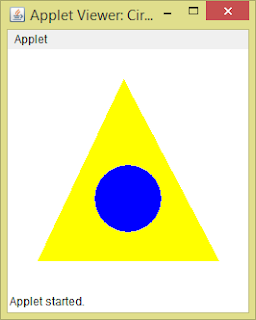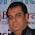### Program to design an applet which draws a circle (having color BLUE) inside a triangle (having color YELLOW)

Given program will draw a circle inside a triangle. The color of circle is "blue" and triangle having color "yellow". To fill circle with respective colours first we have to set the colour using setColor(Color c) method of Graphics class and then use the fillPolygon() and fillOval( ) method.

The fillPolygon( ) method Fills a closed polygon defined by arrays of x and y coordinates. The syntax for fillPolygon( ) is given below:

``void fillPolygon (int[ ] xPoints, int[ ] yPoints, int nPoints)``
```The fillOval( ) method fills an oval bounded by the specified rectangle with the current color. The rectangle is filled using the graphics context's current color. The syntax for fillOval( ) is given below: void fillOval (int x, int y, int width, int height)PROGRAMimport java.applet.Applet; import java.awt.Color; import java.awt.Graphics; public class CircleInsideTriangle extends Applet { public void paint(Graphics g) { // Draws a triangle int xPoints[] = {120, 220, 30}; int yPoints[] = {30, 220, 220}; g.setColor(Color.YELLOW); g.fillPolygon(xPoints, yPoints, 3); // Draws a circle g.setColor(Color.BLUE); g.fillOval(90, 120, 70, 70); } } /* <applet code="CircleInsideTriangle" width=250 height=250> </applet> */OUTPUTC:\>javac CircleInsideTriangle.javaC:\>appletviewer CircleInsideTriangle.java```
``` (adsbygoogle = window.adsbygoogle || []).push({}); ```
``` Applet Get link Facebook Twitter Pinterest Email Other Apps ```
``` ```
``` Comments (function(){/* Copyright The Closure Library Authors. SPDX-License-Identifier: Apache-2.0 */ var m=this||self,p=function(a,b){function d(){}d.prototype=b.prototype;a.superClass_=b.prototype;a.prototype=new d;a.prototype.constructor=a;a.base=function(f,e,q){for(var r=Array(arguments.length-2),n=2;n<arguments.length;n++)r[n-2]=arguments[n];return b.prototype[e].apply(f,r)}},t=function(a){return a};function v(a,b){if(Error.captureStackTrace)Error.captureStackTrace(this,v);else{var d=Error().stack;d&&(this.stack=d)}a&&(this.message=String(a));void 0!==b&&(this.cause=b)}p(v,Error);v.prototype.name="CustomError";function w(a,b){a=a.split("%s");for(var d="",f=a.length-1,e=0;e<f;e++)d+=a[e]+(e<b.length?b[e]:"%s");v.call(this,d+a[f])}p(w,v);w.prototype.name="AssertionError";var x=function(a,b){throw new w("Failure"+(a?": "+a:""),Array.prototype.slice.call(arguments,1));};var B;var D=function(a,b){this.privateDoNotAccessOrElseTrustedResourceUrlWrappedValue_=b===C?a:""};D.prototype.toString=function(){return this.privateDoNotAccessOrElseTrustedResourceUrlWrappedValue_+""};var C={};/* SPDX-License-Identifier: Apache-2.0 */ function F(a,b){if(b instanceof D&&b.constructor===D)b=b.privateDoNotAccessOrElseTrustedResourceUrlWrappedValue_;else{var d=typeof b;x("expected object of type TrustedResourceUrl, got '"+b+"' of type "+("object"!=d?d:b?Array.isArray(b)?"array":d:"null"));b="type_error:TrustedResourceUrl"}a.src=b;var f,e;(f=(b=null==(e=(f=(a.ownerDocument&&a.ownerDocument.defaultView||window).document).querySelector)?void 0:e.call(f,"script[nonce]"))?b.nonce||b.getAttribute("nonce")||"":"")&&a.setAttribute("nonce", f)};function G(a){if("string"!==typeof a)throw Error("Expected a string");if(void 0===B){var b=null;var d=m.trustedTypes;if(d&&d.createPolicy)try{b=d.createPolicy("goog#html",{createHTML:t,createScript:t,createScriptURL:t})}catch(f){m.console&&m.console.error(f.message)}B=b}a=(b=B)?b.createScriptURL(a):a;return new D(a,C)};var L=function(){var a=document;var b="SCRIPT";"application/xhtml+xml"===a.contentType&&(b=b.toLowerCase());return a.createElement(b)};var M=function(a,b,d){var f=null;a&&0<a.length&&(f=parseInt(a[a.length-1].timestamp,10)+1);var e=null,q=null,r=void 0,n=null,y=(window.location.hash||"#").substring(1),H,I;/^comment-form_/.test(y)?H=y.substring(13):/^c[0-9]+\$/.test(y)&&(I=y.substring(1));var Q={id:d.postId,data:a,loadNext:function(k){if(f){var h=d.feed+"?alt=json&v=2&orderby=published&reverse=false&max-results=50";f&&(h+="&published-min="+(new Date(f)).toISOString());window.bloggercomments=function(z){f=null;var u=[];if(z&&z.feed&& z.feed.entry)for(var c,J=0;c=z.feed.entry[J];J++){var l={},g=/blog-(\d+).post-(\d+)/.exec(c.id.\$t);l.id=g?g:null;a:{g=void 0;var K=c&&(c.content&&c.content.\$t||c.summary&&c.summary.\$t)||"";if(c&&c.gd\$extendedProperty)for(g in c.gd\$extendedProperty)if("blogger.contentRemoved"==c.gd\$extendedProperty[g].name){g='<span class="deleted-comment">'+K+"</span>";break a}g=K}l.body=g;l.timestamp=Date.parse(c.published.\$t)+"";c.author&&c.author.constructor===Array&&(g=c.author)&&(l.author={name:g.name? g.name.\$t:void 0,profileUrl:g.uri?g.uri.\$t:void 0,avatarUrl:g.gd\$image?g.gd\$image.src:void 0});c.link&&(c.link&&(l.link=l.permalink=c.link.href),c.link&&(g=/.*comments\/default\/(\d+)\?.*/.exec(c.link.href))&&g&&(l.parentId=g));l.deleteclass="item-control blog-admin";if(c.gd\$extendedProperty)for(var A in c.gd\$extendedProperty)"blogger.itemClass"==c.gd\$extendedProperty[A].name?l.deleteclass+=" "+c.gd\$extendedProperty[A].value:"blogger.displayTime"==c.gd\$extendedProperty[A].name&& (l.displayTime=c.gd\$extendedProperty[A].value);u.push(l)}f=50>u.length?null:parseInt(u[u.length-1].timestamp,10)+1;k(u);window.bloggercomments=null};var E=L();E.type="text/javascript";F(E,G(h+"&callback=bloggercomments"));document.getElementsByTagName("head").appendChild(E)}},hasMore:function(){return!!f},getMeta:function(k,h){return"iswriter"==k?h.author&&h.author.name==d.authorName&&h.author.profileUrl==d.authorUrl?"true":"":"deletelink"==k?d.baseUri+"/delete-comment.g?blogID="+d.blogId+"&postID="+ h.id:"deleteclass"==k?h.deleteclass:""},onReply:function(k,h){null==e&&(e=document.getElementById("comment-editor"),null!=e&&(n=e.style.height,e.style.display="block",q=e.src.split("#")));e&&k&&k!==r&&(document.getElementById(h).insertBefore(e,null),h=q+(k?"&parentID="+k:""),q&&(h=h+"#"+q),e.src=h,e.style.height=n||e.style.height,r=k,e.removeAttribute("data-resized"),e.dispatchEvent(new Event("iframeMoved")))},rendered:!0,initComment:I,initReplyThread:H,config:{maxDepth:d.maxThreadDepth}, messages:b};a=function(){if(window.goog&&window.goog.comments){var k=document.getElementById("comment-holder");window.goog.comments.render(k,Q)}};window.goog&&window.goog.comments?a():(window.goog=window.goog||{},window.goog.comments=window.goog.comments||{},window.goog.comments.loadQueue=window.goog.comments.loadQueue||[],window.goog.comments.loadQueue.push(a))},N=["blogger","widgets","blog","initThreadedComments"],O=m;Nin O||"undefined"==typeof O.execScript||O.execScript("var "+N); for(var P;N.length&&(P=N.shift());)N.length||void 0===M?O=O[P]&&O[P]!==Object.prototype[P]?O[P]:O[P]={}:O[P]=M;}).call(this); blogger.widgets.blog.initThreadedComments( null, null, {});high technologies solutions24 June 2021 at 11:07Thanks for sharing this amazing post this is the content i really looking for, it's very helpful i hope you will continue your blogging anyway if anyone looking for AutoCAD training institute in delhi contact us +91-9311002620 visit-https://www.htsindia.com/autocad-training-institute ReplyDeleteRepliesReplycodingbytes29 July 2021 at 13:52I am appreciative of this blog's ability to provide information on such an important subject about Aws Certification in Delhi. I discovered other segments here, and I'm excited to put these new instructions to use. ReplyDeleteRepliesReplyLaura Bush18 August 2021 at 15:28You've written an excellent post, and you've shared it with us about Software Testing Training Courses Online. Your article provided me with some unique and useful knowledge. I appreciate you sharing this text with us. ReplyDeleteRepliesReplyAdd commentLoad more... Post a Comment BLOG_CMT_createIframe('https://www.blogger.com/rpc_relay.html'); ```
``` ```
``` ```
``` Popular posts from this blog Program to define a class 'employee' with data members as empid, name and salary. Accept data for 5 objects using Array of objects and print it. November 25, 2016 This program demonstrates you the array of objects.  Array of object  is the collection of objects of the same class. The given syntax will define an array of reference therefore assign object to individual reference in the array to declare the array of object. The syntax to define array of object is: <class-name> <array-name> [size]; For example, the following statement will define an array of object having size 6 references to the class  Box . Box b; For creating an array of objects (allocating memory) you have to write down the following statements for (int i=0; i<6; i++) { b[i] = new Box(); // Assigning object to individual reference in the array. } PROGRAM import java.util.Scanner; public class Employee { int empid; String name; float salary; public void getInput() { Scanner in = new Scanner(System.in); System.out.print("Enter the empid :: "); empid = in.nextInt(); System.out.print("Enter the name :: "); Read more Program to input age from user and throw user-defined exception if entered age is negative November 29, 2016 An  Exception  is an abnormal condition that arises in a code sequence at run time. Exceptions are run time errors. Exceptions generated by the Java are related to the fundamental errors that violate the Java language constraints. All exception classes are subtypes of the  java.lang.Exception  class. The exception class is a subclass of the  Throwable  class. Java defines several exception classes inside the standard package  java.lang . Some examples of exceptions are  ArithmeticException, ArrayIndexOutOfBoundsException, IndexOutOfBoundsException, NullPointerException, NumberFormatException, ClassNotFoundException,etc. When Java Interpreter caught an error such as divide by zero, the interpreter creates an exception object and throws it to inform that an error has occurred. Java has its own exception handling mechanism. It performs the following task: Find the problem –  Hit  the exception Inform that an error has occurred –  Throw  the exception Receives the error infor Read more Define a class Student with four data members such as name, roll no.,sub1, and sub2. Define appropriate methods to initialize and display the values of data members. Also calculate total marks and percentage scored by student. November 24, 2016 In this program, first we first create a class  Student  which have data members  name, roll_no, sub1, sub2  and one method to read data here  getdata( )  method and show the result here  show( )  method. We have used  java.io.BufferedReader  class to read data from keyboard at runtime. Then we have created an object of Student class in  StudentDemo  class and called the methods of Student class. When you compile the code using  javac StudentDemo.java  command two .class files will be created which are  Student.class  and  StudentDemo.class , this has the advantage that you can reuse your .class file somewhere in other projects without compiling the code again. You can create as many classes as you want but writing many classes in a single file is not recommended as it makes code difficult to read rather you can create single file for every class. You can also group classes in packages for easily managing your code. PROGRAM import java.lang.*; import java.io.*; class Student { Read more ```
``` ```